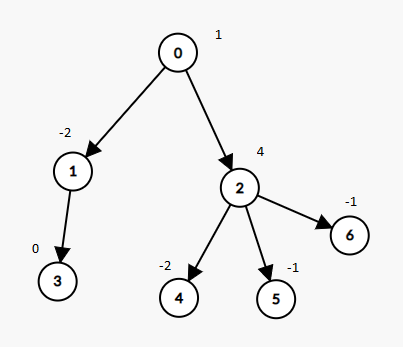All contents and pictures on this website come from the Internet and are updated regularly every week. They are for personal study and research only, and should not be used for commercial purposes. Thank you for your cooperation.

# 1273. Delete Tree Nodes

A tree rooted at node 0 is given as follows:

• The number of nodes is `nodes`;
• The value of the `i`-th node is `value[i]`;
• The parent of the `i`-th node is `parent[i]`.

Remove every subtree whose sum of values of nodes is zero.

After doing so, return the number of nodes remaining in the tree.

Example 1:```Input: nodes = 7, parent = [-1,0,0,1,2,2,2], value = [1,-2,4,0,-2,-1,-1]
Output: 2
```

Constraints:

• `1 <= nodes <= 10^4`
• `-10^5 <= value[i] <= 10^5`
• `parent.length == nodes`
• `parent == -1` which indicates that `0` is the root.

Medium

Prime

Microsoft

### Problem Solution

1273-Delete-Tree-Nodes